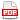﻿ ToposPro » Modules
Modules

Dirichlet

Constructs Voronoi polyhedra of atoms and computes a lot of their geometrical and topological parameters.
• constructs Voronoi polyhedra (VPs) of atoms in crystal structures taking into account both all basis atoms and an arbitrary subset of atomic sublattices specified by the user;
• draws VPs, the corresponding Delaunay stars, coordination polyhedra and Schlegel projections;
• calculates all main geometric parameters of VPs;
• determines topological parameters of VPs and automatically forms the library of VP topological types;
• calculates VPs for a group of crystal structures and forms data sets for the subsequent statistical analysis;
• computes parameters for estimating empty space in the crystal structure;
• searches for the possible migration paths of mobile ions.
In the Dirichlet program a very fast "gift wrapping" algorithm of VP construction has been realized for the first time. This algorithm allows one to calculate millions of VPs in a reasonable time.
IsoCryst

Draws various images of crystal structures and provides their comprehensive geometrical analysis.
• constructs stick, sphere-and-stick and polyhedral images of crystal structures and provides convenient services for their rotation, scaling and editing;
• computes geometrical characteristics of crystal structure (interatomic distances, bond and torsion angles, RMS planes, etc.);
• constructs Voronoi and coordination polyhedra;
• analyzes packings of polymeric or molecular structural units;
• searches for finite fragments in an infinite crystal structure.
HSite

Determines positions of hydrogen atoms, which have not been localized in crystal-structural experiment.

The program HSite uses unique algorithms, which allow it to determine the hydrogen positions not only for organic molecules, but also for inorganic and metal-organic structures.
IsoTest

Automatically tests crystal structures on isotypism (topological similarity) and determines the degree of their similarity.
• automatically tests crystal structures on isotypism (topological similarity) and determines the degree of their similarity;
• provides topological analysis of atomic sublattices and detects close packings at any degree of their distortion;
• automatically classifies crystal structures according to the structure type concept.
The program IsoTest uses the adjacency matrix built by the AutoCN program, and the analytical algorithms of the ADS program.

AutoCN

Computes the adjacency matrix of crystal structure, determines types of interatomic interactions (valence, H-bond, specific, van der Waals).
• computes the adjacency matrix of crystal structure;
• determines valence interatomic interactions, hydrogen bonds, specific and van der Waals contacts;
• computes geometrical parameters of interatomic interactions.
The program AutoCN uses Voronoi polyhedra and a number of special algorithms to build the adjacency matrix. The matrix is stored in the database as a weighted colored labeled quotient graph.
StatPack

Realizes a number of standard statistical methods for processing the data obtained with the Dirichlet, ADS and DiAn programs.
• performs statistical analysis for geometrical parameters of Voronoi polyhedra as well as of their faces, edges and vertices;
• builds 1D, 2D, and 3D distributions of geometrical parameters of atoms or their Voronoi polyhedra;
• detects errors in crystallographic data.Certificate

Computes topological parameters of atomic and molecular networks, performs topological classification, determines structural groups, builds tilings.
• determines structural groups (atoms, ligands, molecules, clusters, rods, layers, frameworks) and simplifies the initial atomic network to an underlying net;
• determines the topology of structural groups and the coordination types of ligands;
• computes molecular coordination numbers and analyzes topology of molecular packings;
• computes topological indices for the crystal structure network;
• assigns topological type to the underlying net and builds the TTD collection;
• detects different kinds of entanglements (interpenetration, polycatenation, polythreading, self-catenation) and classifies them;
• builds tiles and tilings, determines topological types of tiles and builds the library of the topological types of tiles.
The program ADS uses the adjacency matrix computed by the AutoCN program and realizes the representation of a crystal structure as a finite quotient graph. This approach allows one to store and analyze the topology of a crystal structure as a whole.
DiAn

Computes interatomic distances, bond angles and geometrical fingerprints for crystal structures.
• computes interatomic distances and bond angles for a set of atoms;
• computes radial and angular fingerprints of atoms in the crystal structure;
• determines the type of the coordination figure of the atom;
• builds data sets with geometrical parameters for the subsequent statistical analysis by the program StatPack.